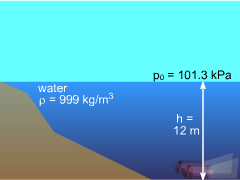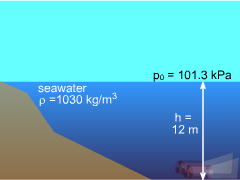Ch 2. Fluid Statics Multimedia Engineering Fluids PressureVariation PressureMeasurement HydrostaticForce(Plane) HydrostaticForce(Curved) Buoyancy
 Chapter 1. Basics 2. Fluid Statics 3. Kinematics 4. Laws (Integral) 5. Laws (Diff.) 6. Modeling/Similitude 7. Inviscid 8. Viscous 9. External Flow 10. Open-Channel Appendix Basic Math Units Basic Equations Water/Air Tables Sections Search eBooks Dynamics Fluids Math Mechanics Statics Thermodynamics Author(s): Chean Chin Ngo Kurt Gramoll ©Kurt GramollFLUID MECHANICS - CASE STUDY SOLUTIONProblem Diagram I By assuming the fluid is incompressible and at rest, the hydrostatic pressure distribution is expressed as:      p1 = ρgh + p0 From standard handbooks, it is noted that the atmospheric pressure at the sea level is p0 = 101.3 kPa and the acceleration of gravity is g = 9.8 m/s2. At fresh water temperature of 16oC, the density of water = 999 kg/m3 and the density of seawater = 1030 kg/m3.Problem Diagram II The absolute pressure at a depth of 12 meters of water is      p1 = (999 kg/m3) (9.8 m/s2) (12 m) + 101.3 kPa          = 219 kPa whereas for seawater:      p1 = (1030 kg/m3) (9.8 m/s2) (12 m) + 101.3 kPa          = 222 kPa Therefore, it can be concluded that the density of the fluid (fresh water vs. seawater) in this scenario plays a very small role (1%) in the design criteria.

Practice Homework and Test problems now available in the 'Eng Fluids' mobile app
Includes over 250 problems with complete detailed solutions.
Available now at the Google Play Store and Apple App Store.